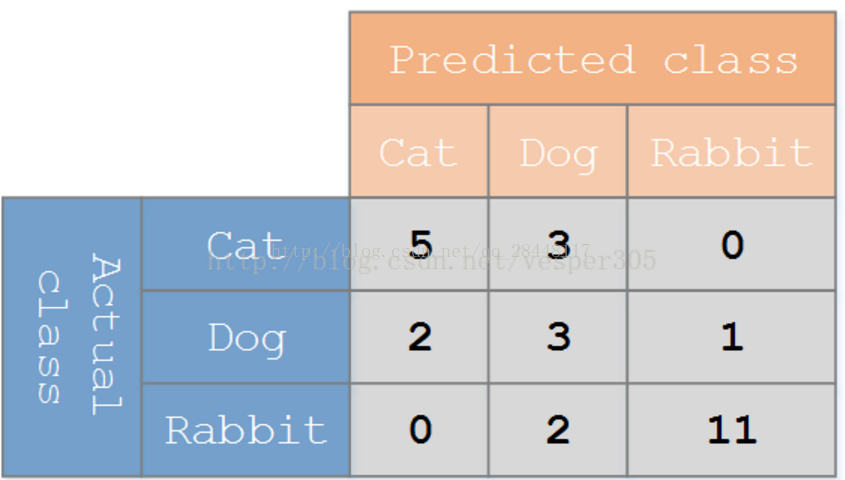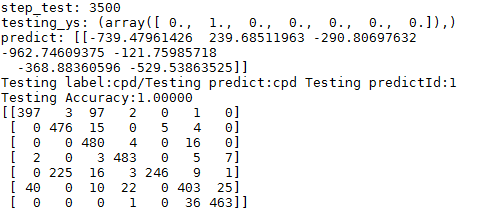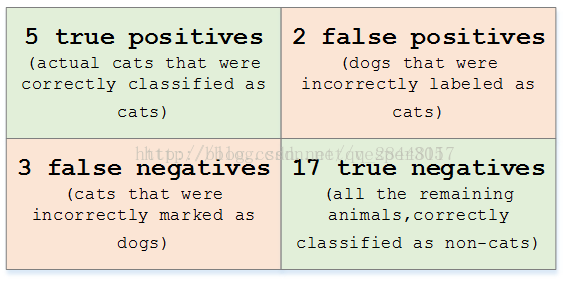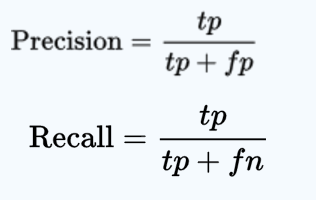# confusion_matrix（混淆矩阵）

2018/08/09 18:01

## 混淆矩阵# tf.confusion_matrix

``````tf.confusion_matrix(
labels,
predictions,
num_classes=None,
dtype=tf.int32,
name=None,
weights=None
)``````

#### ARGS：

• `labels``Tensor`分类任务的1-D 真实标签。
• `predictions``Tensor`给定分类的1-D 预测。
• `num_classes`：分类任务可能具有的标签数量。如果未提供此值，则将使用预测和标签数组计算该值。
• `dtype`：混淆矩阵的数据类型。
• `name`：范围名称。
• `weights`：可选`Tensor`的形状匹配`predictions`

#### 返回：

甲`Tensor`类型的`dtype`具有形状`[n, n]`表示所述混淆矩阵，其中`n`是在分类任务可能的标签的数量。

``````tf.confusion_matrix([1, 2, 4], [2, 2, 4]) ==>
[[0 0 0 0 0]
[0 0 1 0 0]
[0 0 1 0 0]
[0 0 0 0 0]
[0 0 0 0 1]]``````

1.定义两个矩阵，用于放真实的标签和预测的标签，大小根据测试的次数计算,全为0.[1,test_num]

``````Y_true=np.zeros(len(test_num))#真实的标签
Y_predict=np.zeros(len(test_num))#预测的标签``````

2.将每步训练的标签放在矩阵中，我的项目中预测的的为标签，类似[0,0,0,1,0,0,0]，取出最大的，即为4，本步在循环中，记录每步训练。

``````Y_true[step_test]=np.argmax(testing_ys)
Y_predict[step_test]=np.argmax(predict)``````

3.调用TensorFlow的混淆矩阵函数，这一步需要将矩阵转换为tensor，在TensorFlow中运行的单元为tensor

``````confuse_martix=sess.run(tf.convert_to_tensor(tf.confusion_matrix(Y_true,Y_predict)))
print(confuse_martix)``````

4.结果# 匹配矩阵https://blog.csdn.net/qq_28448117/article/details/78219549

0
0 收藏

### 作者的其它热门文章0 评论
0 收藏
0